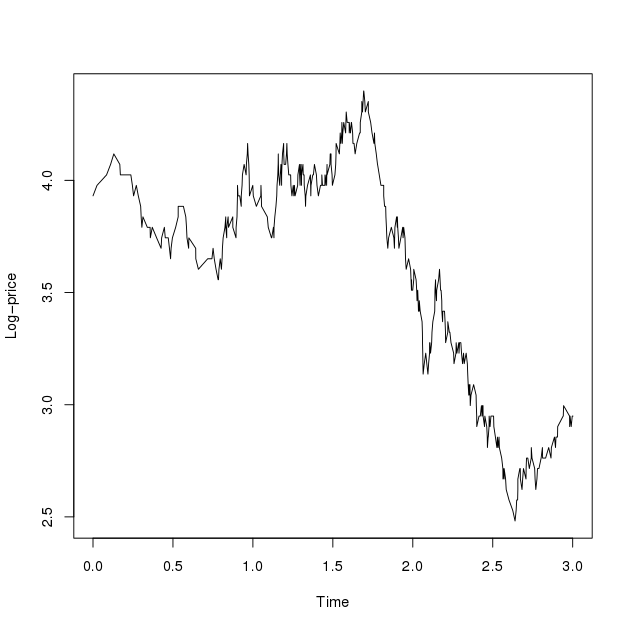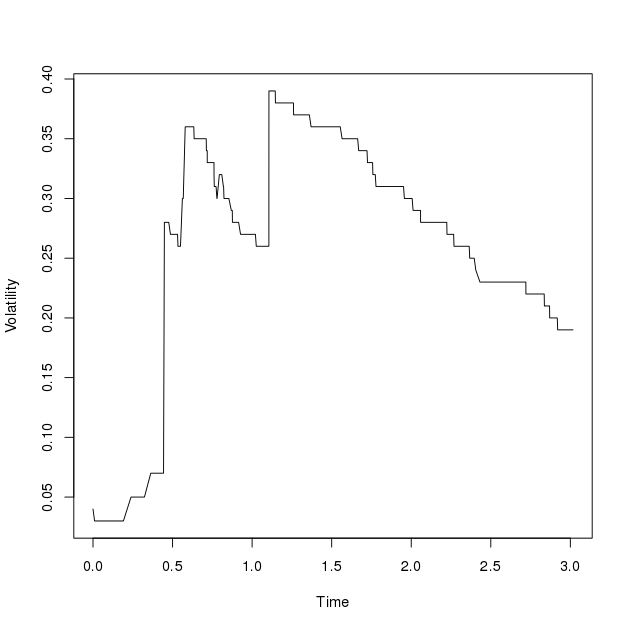# Stochastic Volatility Models

In the previous sections we have only considered price processes where the coefficients in the governing SDE are constant (see section on jump-diffusion models). One can also consider models where the coefficients are functions of time and the stock price.
An alternative approach is to allow the paremeters such as the volatility $$\sigma$$ to be random variables themselves. More precisely, we look at models of the form $$(Y_t, V_t)$$ where the log-price $$Y_t$$ and the volatility $$V_t$$ are governed by different SDEs. These type of models are often called stochastic volatility models and an example of which is the Barndorff-Nielson--Shephard (BNS) model. The BNS model is of the form

\begin{align} \mathrm{d}Y_t &= \Big(r -\delta - \frac{1}{2}V_t\Big)\mathrm{d}t + \sqrt{V_t}\mathrm{d}W_t + \rho\mathrm{d}J_{\lambda t}, \\ \mathrm{d}V_t &= -\lambda V_t \mathrm{d}t + \mathrm{d}J_{\lambda t}, \end{align}

where $$r,\lambda>0$$, $$\rho<0$$, $$(W_t)_{t\geq 0}$$ is a standard Brownian motion and $$(J_{\lambda t})_{t\geq 0}$$ is a pure jump process that increases almost surely and has Levy measure $$\nu$$. $$\delta$$ is a time-independent constant which is chosen so that the discounted asset price $$\tilde{S}_t = \exp(-rt + Y_t)$$ is a martingale. It can be shown that necessarily $$\delta = \lambda\kappa(\rho)$$ where $$\kappa$$ is the cumulant generating function of $$(J_t)_{t\geq 0}$$ given by

$$\kappa(u) = \int_0^\infty (e^{u\xi} - 1) \;\nu(\mathrm{d}\xi).$$

The negative constant $$\rho$$ is there to introduce a correlation between the log-price and the volatility; a jump in the volatility will cause a jump downwards in the log-price. Typically, the jump term $$(J_t)_{t\geq 0}$$ is taken to be a compound Poisson process

$$J_t = \sum_{i=1}^{N_t} U_i$$

where $$(N_t)_{t\geq 0}$$ is a Poisson process with intensity $$\alpha$$ and $$\{U_i\}_{i\in\mathbb{N}}$$ is a sequence of i.i.d. random variables which we assume to be exponentially distributed with mean $$1/\eta$$ and so $$J_{\lambda t}$$ has Levy measure given by $$\nu(\mathrm{d}h) = \alpha\lambda g(h)$$ with $$g(h) = \eta\exp(-\eta h)$$ being the density of a exponentially distributed random variable. For this choice of $$(J_t)_{t\geq 0}$$ a simple integration shows that

$$\kappa(\rho) = \frac{\alpha \rho}{\eta - \rho}$$.

As before option prices are given as expectations of the payoff under the risk neutral measure so Monte Carlo methods can be used.Notice that the BNS model is a two-dimensional model so one needs to approximate it by a Markov chain on a finite two-dimensional state space. In one-dimensional models the Q-matrix $$\Lambda$$ of the approximating chain is a $$N\times N$$ matrix where $$N$$ is the number of grid points. If now we have $$N$$ grid points in bothe the log-price and the volatility component then the Q-matrix is a $$N^2\times N^2$$ matrix. Thus, simulations of these higher dimensional models can be computationally expensive.

As in the jump-diffusion case we calculate the Q-matrix of the Markov chain by considering the jump and the diffusion components of the process separate and compute the corresponding transition rate matrices $$\Lambda_J$$ and $$\Lambda_D$$, then $$\Lambda$$ is defined as the sum $$\Lambda$$ = $$\Lambda_D + \Lambda_J$$.

### Diffusion Matrix

We can express the diffusion part of the BNS model in vector form as

$$\mathrm{d}\begin{pmatrix} Y_t \\ V_t \end{pmatrix} = \begin{pmatrix} r- \delta - \frac{1}{2}V_t \\ -\lambda V_t \end{pmatrix}\mathrm{d}t + \begin{pmatrix} \sqrt{V_t} \\ 0 \end{pmatrix}\mathrm{d}W_t$$

which provides the infinitesimal generator $$\mathcal{L}_D$$ as

$$\mathcal{L}_Df(y,v) = \Big(r - \delta - \frac{1}{2}v\Big)\frac{\partial f}{\partial y}(y,v) - \lambda v\frac{\partial f}{\partial v}(y,v) + \frac{1}{2}v\frac{\partial^2 f}{\partial y^2}(y,v).$$

where $$f \in C_0^2 (\mathbb R ^2)$$ the space of twice differentiable functions such that $$\lim_{|x|\to\infty}f(x) = 0$$. One can discretize this PDE by finite differences using either forward or backward difference for the first order derivatives:

$$\frac{1}{h}\Big( f(y + h,v) - f(y,v)\Big)\quad\text{or}\quad \frac{1}{h}\Big( f(y,v) - f(y - h, v)\Big)$$

The choice of which one to use is determined by the condition that the result must be a Q-matrix. For the second order derivative term central difference can be used:

$$\frac{1}{h^2}\Big( f(y + h, v) - 2f(y,v) + f(y - h,v)\Big)$$.

The forward, backward and central differences in the $$v$$ variable are defined similarly. Let $$\mathbb{G} = \{(y_i,v_j) : 1\leq i,j\leq N, y_1 > \cdots > y_N, v_1 < \cdots < v_N\}$$ be a grid with grid spacing $$h_v$$ in the volatility component and grid spacing $$h_y = |\rho|h_v$$ in the log-price component. The choice of the grid spacing $$h_y$$ is so that a jump in the volatility of one grid point translates to a jump of one grid point in the log-price and so on. Now denote $$f(y_i,v_j)$$ by $$f_{ij}$$ then the discretisation of $$\Lambda_D$$ is

$$\frac{1}{h_y}\Big(\mu f_{i-1j} - \mu f_{ij} - \frac{1}{2}v_jf_{ij} + \frac{1}{2}v_jf_{i+1}j\Big) + \frac{1}{h_v}\Big(\lambda v_jf_{ij-1} - \lambda v_jf_{ij}\Big) + \frac{1}{h_y^2}\Big(\frac{1}{2}v_jf_{i-1j} - v_jf_{ij} + \frac{1}{2}v_jf_{i+1j}\Big)$$

It is easy to see that the above equation can be expressed as a matrix-vector product $$\Lambda_J \mathbf{f}$$ where $$\mathbf{f} = \big(f_{11},f_{12},\ldots,f_{1N},f_{21},\ldots,f_{NN}\big)^T$$ and the $$\Lambda_J$$ is the desired discretisation.

### Jump Matrix

In general, the generator of a compound Poisson process on $$\mathbb{R}^d$$ is given by

$$\mathcal{L}_J f(x) = \int_{\mathbb{R}^d} \big(f(x+h) - f(x)\big)\;\nu(\mathrm{d}h)$$,

where $$f \in C_0(\mathbb{R}^d)$$. In the BNS model, since the log-price process and the volatility are both driven by the same jump process with correlation $$\rho < 0$$ we can write the generator $$\mathcal{L}_J$$ for the jump component as

$$\mathcal{L}_Jf(y,v) = \int_{\mathbb{R}} \big( f(y + \rho h, v + h) - f(y,v) \big)\;\nu(\mathrm{d}h),$$

The above integral can be approximated by Riemann sums on the grid $$\mathbb{G}$$,

\begin{align*}\mathcal{L}_J f(y_i,v_j) &\approx \sum_{n=1}^{N - (i\vee j)} \Big( f(y_i + n\rho h_v,v_j + nh_v) - f(y_i,v_j) \Big) \alpha\lambda g(h_v)nh_v \\ &= \sum_{n=1}^{N - (i\vee j)} \Big( f(y_{i + n} ,v_{j + n}) - f(y_i,v_j) \Big) \alpha\lambda g(h_v)nh_v \end{align*}.

Again the above can be written as a matrix-vector product $$\Lambda_J\mathbf{f}$$ where $$\mathbf{f}$$ is as above.The above graphs show a sample path of the log-price and the volatility obtained from the approximating Markov chain. A grid of size 100 $$\times$$ 100 was used to generate the path. Notice that the log-price looks like a typical jump-diffusion process while the volatility maoves up entirely by jumps and slowly drifts to zero in the abscence of jumps due to the negative term in the governing SDE.